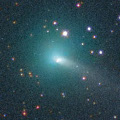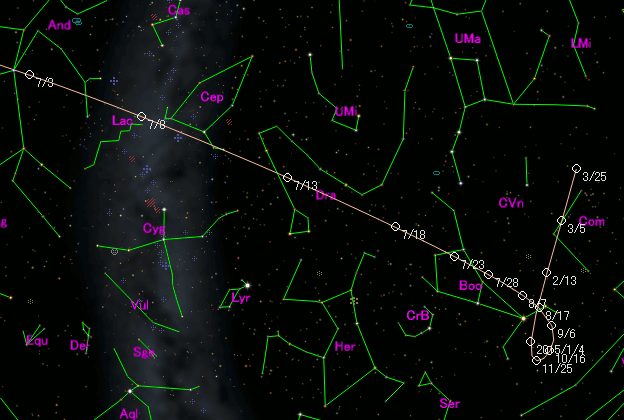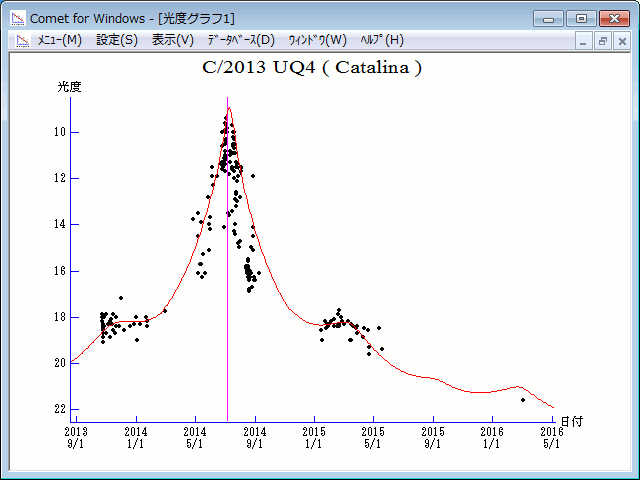# \$B%+%?%j%JWB@1(B

C/2013 UQ4 ( Catalina )###\$B%W%m%U%#!<%k(B

 \$BH/8+F|(B 2013\$BG/(B10\$B7n(B23\$BF|(B \$BH/8+8wEY(B 18.4\$BEy(B \$BH/8+ J. A. Johnson (Catalina Sky Survey)

###\$B###\$B50F;MWAG(B

```Epoch 2014 July 2.0 TT = JDT 2456840.5
T 2014 July 5.95957 TT                                  MPCW
q   1.0809747            (2000.0)            P               Q
z  +0.0165103      Peri.   23.31199     +0.45982753     -0.80077918
+/-0.0000002      Node   317.66224     -0.87772704     -0.47543744
e   0.9821528      Incl.  145.25840     -0.13473635     +0.36429651
From 1627 observations 2013 Oct. 23-2016 Mar. 3, mean residual 0".5.
```

###\$B@1?^(B###\$B8wEYJQ2=(B

```        H = 12.8  G = 0.15                [    ,-136]  (              \$B!A(B2014\$BG/(B 2\$B7n(B19\$BF|(B)
m1 = 11.0 + 5 log\$B&\$(B + 12.5 log r  [-136, 130]  (2014\$BG/(B 2\$B7n(B19\$BF|!A(B2014\$BG/(B11\$B7n(B12\$BF|(B)
H = 12.8  G = 0.15                [ 130,    ]  (2014\$BG/(B11\$B7n(B12\$BF|!A(B              )
```##### \$B50F;MWAG\$O(BM.P.E.C. 2016-F43\$B\$K7G:\\$5\$l\$?\$b\$N\$G\$9!#(B \$B@1?^\$O%9%F%i%J%S%2!<%?(B Ver.8 (\$B%"%9%H%m%"!<%D(B) \$B\$G:n@.\$7\$?\$b\$N\$G\$9!#(B \$B8wEY%0%i%U\$O(BComet for Windows\$B\$G:n@.\$7\$?\$b\$N\$G\$9!#(B